# C++多态性总结

## 二，多态性分类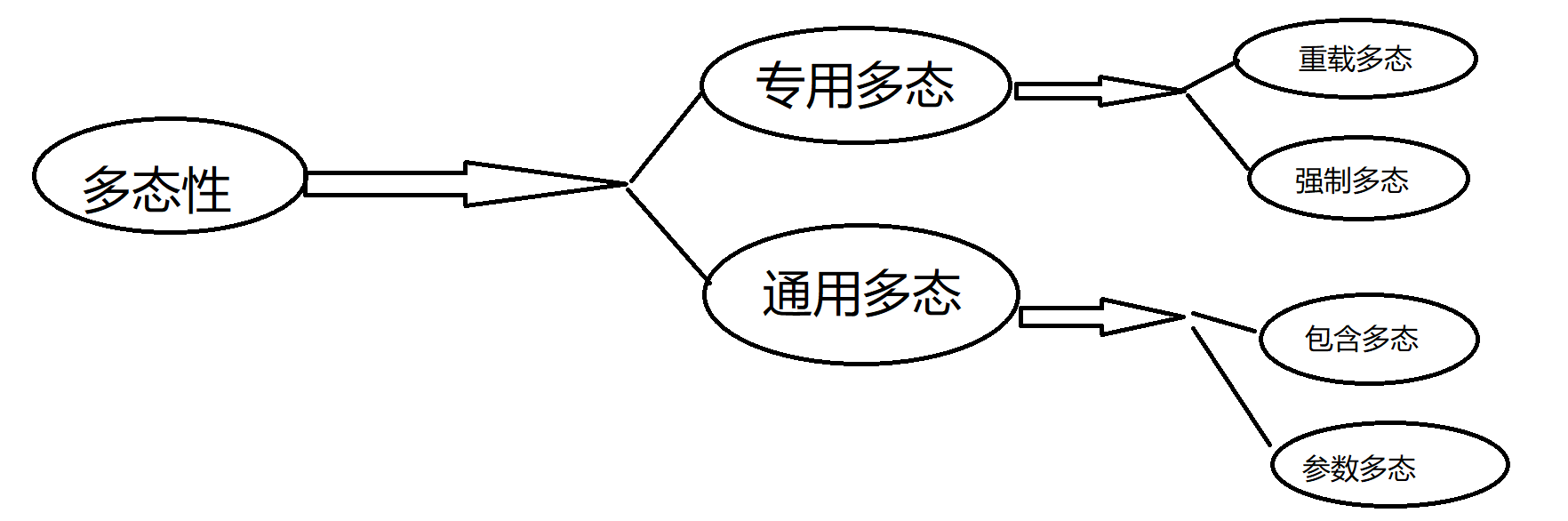## 1，重载多态：

int add(int x, int y) {
return x + y;
}
double add(double x, double y) {
return x + y;
}
float add(float x, float y) {
return x + y;
}


## 重载为成员函数：

{

}

#include<iostream>
using namespace std;
class Complex {
public:
Complex(double r = 0, double i = 0) :real(r), image(i) {}

Complex& operator ++() {
++this->image;
++this->real;
return (*this);

};//前++

Complex operator ++(int) {
Complex temp = *this;
++(*this);//调用前置++
return temp;
};//后++

void diaplayComplex() const {
cout << "(" << this->real << "," << this->image << ")" << endl;
};
private:
double real;
double image;

};

int main()
{
Complex c1(1, 2);
Complex c3(1, 2);
Complex c2;
c2=c1++;
c1.diaplayComplex();
c2.diaplayComplex();

c2=++c3;
c3.diaplayComplex();
c2.diaplayComplex();
return 0;
}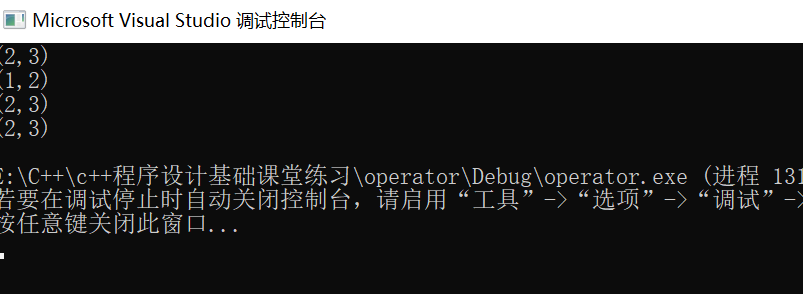#include"operator.h"
#include <iostream>
using namespace std;
class Complex {
public:
Complex(double r = 0, double i = 0) :real(r), image(i) {}
Complex operator +(const Complex &c) const;
void diaplayComplex() const;
private:
double real;
double image;

};
Complex Complex::operator +(const Complex &c)const {
return Complex::Complex(c.real + this->real, c.image + this->image);
};
void Complex::diaplayComplex() const {
cout << "(" << this->real << "," << this->image << ")" << endl;
};
int main()
{
Complex c1(1,2);
Complex c2(1, 2);
Complex c3;//保存运算结果
Complex c4;//保存运算结果
c3 = c1.operator+(c2);//传统方法调用
c3.diaplayComplex();
c4 = c1 + c2;//易懂的方法调用
c4.diaplayComplex();
return 0;
}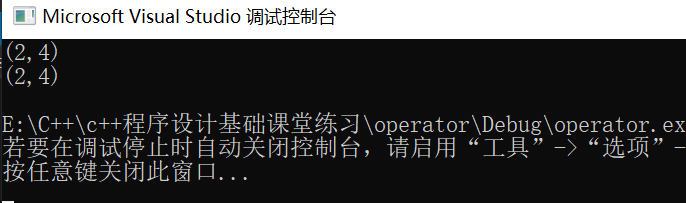## 重载为非成员函数：

{

}

//对类Point重载“++”(自增),"--"（自减）运算符，要求同时重载前缀和后缀的形式
#include "pch.h"
#include <iostream>
using namespace std;

class Point {
public:
Point(int x=0, int y=0) :x(x), y(y) {}
void showPoint();
//以非成员函数类型实现
friend Point& operator ++( Point&  p);
friend Point operator ++(Point& p, int);

private:
int x, y;
};
void Point::showPoint() {
cout << "(" << x << "," <<y<< ")" << endl;
}
Point& operator ++( Point &p) {//前置++
++(p.x);
++(p.y);
return p;
};
Point operator ++(Point& p, int) {//后置++
Point t = p;
++p;
return t;
};

int main()
{
Point p1, p2, p3;
p3 = p1++;
p3.showPoint();
p1.showPoint();
cout << "------------------------------------------" << endl;
p3 = ++p2;
p3.showPoint();
p2.showPoint();
return 0;
}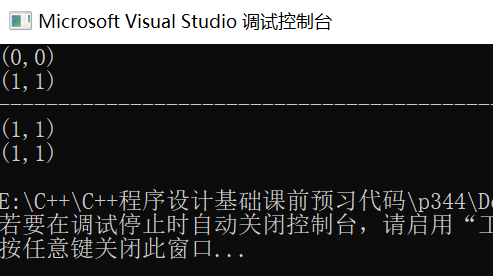## 2,强制多态

int a=1;
float b=2.4f;
float re=a+b;


## 包含多态的条件：

//编写一个哺乳动物类Mammal，再由此派生出狗类Dog,Cat类，三者都声明speak()成员函数，该函数在基类中被声明为虚函数。

#include <iostream>
#include<string >
using namespace std;
class Mammal {
public:
virtual void speak();
private:
string name;
};
class Dog :public Mammal{
public:
virtual void speak();
private:
int age;

};
class Cat :public Mammal {
public:
virtual void speak();
private:
int age;

};
void fun(Mammal *m) {//通过传入不同对象的实参，可调用产生对应的成员函数，以实现多态的功能
m->speak();
}
void Mammal::speak() { cout << "I don't know what to speak" << endl; };
void Dog::speak() { cout << "wang ~~~wang~~~ wang~~~" << endl; };
void Cat::speak() { cout << "miao ~~~miao~~~ miao~~~" << endl; };
int main()
{
Dog d;
Cat c;
//传入不同对象的参数，实现相对应的功能（多态）
fun(&d);
fun(&c);

return 0;
}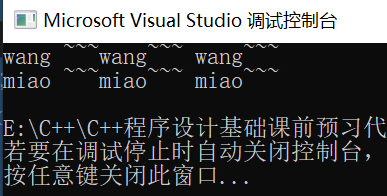void fun(Mammal *m) {//通过传入不同对象的实参，可调用产生对应的成员函数，以实现多态的功能
m->speak();
}


void fun(Mammal &m) {//通过传入不同对象的实参，可调用产生对应的成员函数，以实现多态的功能
m.speak();
}


## 纯虚函数与抽象类：

virtual 函数类型 函数名 （参数表）=0；

//编写一个哺乳动物类Mammal，再由此派生出狗类Dog,Cat类，三者都声明speak()成员函数，该函数在基类中被声明为虚函数。

#include <iostream>
#include<string >
using namespace std;
class Mammal {
public:
virtual void speak()=0;
private:
string name;
};
class Dog :public Mammal{
public:
virtual void speak();
private:
int age;

};
class Cat :public Mammal {
public:
virtual void speak();
private:
int age;

};
void fun(Mammal &m) {//通过传入不同对象的实参，可调用产生对应的成员函数，以实现多态的功能
m.speak();
}
void Dog::speak() { cout << "wang ~~~wang~~~ wang~~~" << endl; };
void Cat::speak() { cout << "miao ~~~miao~~~ miao~~~" << endl; };
int main()
{
Dog d;
Cat c;
//传入不同对象的参数，实现相对应的功能（多态）
fun(d);
fun(c);

return 0;
}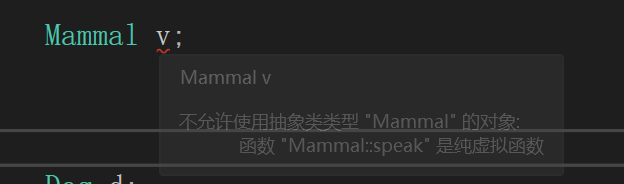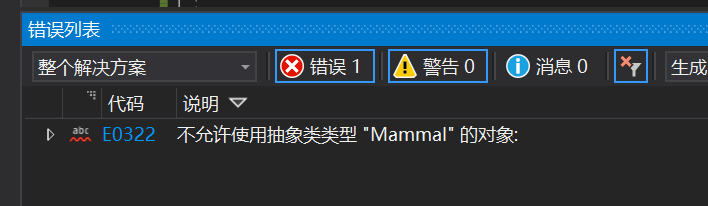## 4，参数多态

template <模板参数表>

{

}

#include "pch.h"
#include <iostream>
using namespace std;
template <typename T>
T abs(T x)
{
return x < 0 ? -x : x;
}

int main() {
int a = 1;
double b = -1;
cout << abs(a) << endl;
cout << abs(b) << endl;
return 0;
}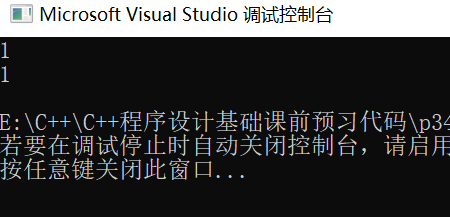## 四，总结

posted @ 2019-10-26 14:31  --believe  阅读(5753)  评论(0编辑  收藏  举报